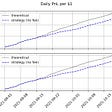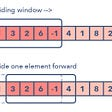# Ugly Number Problems on LeetCode

The problems on leetcode are listed below, and the number of stars indicate the difficulty of each problem.

## Definition and Analysis

Ugly numbers are positive numbers whose prime factors only include `2, 3, 5` . However, sometimes this definition can be extended to a list of prime factors `pl`, such as [3, 5, 7], [2, 3, 7, 11]. This means an ugly number can be represented as:

`u=(2^i)*(3^j)*(5^k)`

There are different types of questions involved with ugly number:

• Check if a number is an ugly number.
• Find the n-th ugly number.

## Checking

The first question is easy, to check if a number `u`, we start from `u`, keep divide it by any of the prime factors that is divisible until the number `u=1` .

## n-th Ugly Number

To find the n-th ugly number, there are multiple ways to do it.

Method 1: First and the most naive solution is for each prime factor, get `n` top ugly number for each prime factor, and merge them in a set, sort the list and the n-th ugly number will be the n-th number in the list. The complexity will be O(nlgn) and space complexity is O(n). For example:

`n=42: [2, 4, 6, 8]3: [3, 6, 9, 12]5: [5, 10, 15, 20]list = [2, 3, 4, 5, 6, 8, 9, 10, 12, 15, 20]4th will be 5`

Method 2: The second will be more efficient. For each prime factor, we list its current smallest number, at first it will be [2, 3, 5]. Then, we choose the smallest which is 2 from the list, and we put 2*2 inside, the candidates list become [3, 4, 5]. Then we pop 3 out, and supply the candidates with 6, [4, 5, 6].

`n=4, candidates = [2, 3, 5]1-th: 2, put 2+2 inside, candidates =[3, 4, 5]2-th: 3, put 3+3 inside, candidates=[4,5,6]3-th: 4, put 4+2 inside, candidates =[5, 6, 6], 4-th: 5, put 5+5 inside, candidates = [6, 6, 10]5-th: 6, however, there are two situations that both equals to 6, put 6+2 inside, and 6+3, inside, candidates = [8, 9, 10]6-th: 8, put 8+2 inside, candidates = [9, 10, 10] 7-th: 10, put 10+2, 10+5 inside, candidates = [9, 12, 15]`

Imagine the `candidates` list as a buffer, once we take one, we supply each prime factor’s next number to the list. This only require constant number of space, and the time complexity will be O(n). However, when `n is extremely large, this is still not doable as shown in `1201. Ugly Number III(**).

Method 3: What if the first prime factor is so small, and the other prime factors are so large, and that most of the time that we only need to apply the first prime factor. Another approach is we first only use the first smallest prime factor, we build a list of `n` candidates here:

`n = 6,candidates = [2, 4, 6, 8, 10, 12]. The maximum is 12. Now, we check prime factor 3, 3 < 12, and 3 not in candidates, use 3 to replace 12. max_2 = 10, max_3 = 33+3 < 10, and 6 is overlap with previous number, skip. 3+3+3 = 9 < 10, use 9 to replace 10, max_2= 8, max_3=9. `

We need to pay attention, when we are decreasing the maximum one, it might be equal to the other maximum for each factor, then in this case, we have to decrease the other maximum to make sure `max_2 > max_3 > max_5`. The time complexity in this case is `O(p1*n//p2+p1*n//p2).` When p2 and p3 is a lot larger, this will greatly decrease the time complexity.

## Problems

263. Ugly Number

Write a program to check whether a given number is an ugly number.

Ugly numbers are positive numbers whose prime factors only include `2, 3, 5`. For example, `6, 8` are ugly while `14` is not ugly since it includes another prime factor `7`.

Note:

1. `1` is typically treated as an ugly number.
2. Input is within the 32-bit signed integer range.

Solution: num =2^i3^j5^k, keep divide [2,3,5], if the remainder is==0, or else stop. return False

`def isUgly(self, num):        """        :type num: int        :rtype: bool        """        if num==0:            return False        factor =[2,3,5]        for f in factor:            while num%f==0:                num/=f        return num==1`

264. Ugly Number II

Write a program to find the `n`-th ugly number.

Ugly numbers are positive numbers whose prime factors only include `2, 3, 5`. For example, `1, 2, 3, 4, 5, 6, 8, 9, 10, 12` is the sequence of the first `10` ugly numbers.

Note that `1` is typically treated as an ugly number, and n does not exceed 1690.

Two solutions: 1. use a list to save all the first 1690 numbers and sort the list. 2. to generate in time, use three pointers, to record the last result to use each pointer.

`def nthUglyNumber(self, n):        """        :type n: int        :rtype: int        """        # nums=[]        # for i in range(int(log(1690,5))):        #     for j in range(int(log(1690,3))):        #         for k in range(int(log(1690,2))):        #             nums.append((5**i)*(3**j)*(2**k))        # nums.sort()        # print(len(nums))        # print(nums)                nums=        idx_2, idx_3, idx_5 = 0, 0, 0        for i in range(n-1):            next2=nums[idx_2]*2            next3=nums[idx_3]*3            next5=nums[idx_5]*5            next=min(next2,next3,next5)            nums.append(next)            if next==next2:                idx_2+=1            if next ==next3:                idx_3+=1            if next==next5:                idx_5+=1        return nums[-1]`

313. Super Ugly Number

Write a program to find the nth super ugly number.

Super ugly numbers are positive numbers whose all prime factors are in the given prime list `primes` of size `k`. For example, `[1, 2, 4, 7, 8, 13, 14, 16, 19, 26, 28, 32]` is the sequence of the first 12 super ugly numbers given `primes` = `[2, 7, 13, 19]` of size 4.

Note:
(1) `1` is a super ugly number for any given `primes`.
(2) The given numbers in `primes` are in ascending order.
(3) 0 < `k` ≤ 100, 0 < `n` ≤ 106, 0 < `primes[i]` < 1000.
(4) The nth super ugly number is guaranteed to fit in a 32-bit signed integer.

Solution:the same as the last, except that the primes different

`def nthSuperUglyNumber(self, n, primes):        """        :type n: int        :type primes: List[int]        :rtype: int        """        nums=        idexs=*len(primes) #first is the current idex        for i in range(n-1):            min_v = maxsize            min_j = []            for j, idex in enumerate(idexs):                v = nums[idex]*primes[j]                if v<min_v:                    min_v = v                    min_j=[j]                elif v==min_v:                    min_j.append(j) #we can get mutiple j if there is a tie            nums.append(min_v)            for j in min_j:                idexs[j]+=1         return nums[-1]`

1201. Ugly Number III

Write a program to find the `n`-th ugly number.

Ugly numbers are positive integers which are divisible by `a` or `b` or `c`.

Example 1:

`Input: n = 3, a = 2, b = 3, c = 5Output: 4Explanation: The ugly numbers are 2, 3, 4, 5, 6, 8, 9, 10... The 3rd is 4.`

Example 2:

`Input: n = 4, a = 2, b = 3, c = 4Output: 6Explanation: The ugly numbers are 2, 3, 4, 6, 8, 9, 10, 12... The 4th is 6.`

Example 3:

`Input: n = 5, a = 2, b = 11, c = 13Output: 10Explanation: The ugly numbers are 2, 4, 6, 8, 10, 11, 12, 13... The 5th is 10.`

Example 4:

`Input: n = 1000000000, a = 2, b = 217983653, c = 336916467Output: 1999999984`

Use method 3:

`def nthUglyNumber(self, n: int, a: int, b: int, c: int) -> int:        t = sorted(list(set([a, b, c])))        e = t*n                t_max = [e]+[-float('inf')]*(len(t)-1) # max for each prime factor        max_index = 0        ans = max(t_max)        for j, v in enumerate(t[1::]): # for the next prime factor, replace its number.             i = 1            while True:                temp = i * v # possible ugly number within the range of n.                 if temp >= t_max[max_index]:                    break                if any([temp%d==0 for d in t[:j+1]]): #exist                    i+= 1                    continue                else: # not exist, kick out max                    temp_max =  t_max[max_index] - t[max_index]                        t_max[max_index] = temp_max                    #check if there are other that equals to the max                    for k in range(max_index+1, j+1):                        if t_max[k] == temp_max:                            t_max[k] -= t[k]                    t_max[j+1] = max(t_max[j+1], temp) #update current max                    max_index = t_max.index(max(t_max))                    i+=1return max(t_max)`

--

--

--

## More from Algorithms and Coding Interviews

Sharing methods to solve questions on leetcode, trying to systematize different types of questions

## Recommended from Medium## Six Powerful Quotes That Slapped Me Square in the Face,!## Instrumental Finance: LP Yield Optimization and Portfolio Management## Data Wrangling on the WeRateDogs Twitter Data## The Ultimate Guide to Pricing your SaaS Startup with Data## AI PM and Architect; Continuation of Data Science Unicorn Search## Lessons in My First Year as a Data Scientist## Li Yin

Founder@sylphai.com. AI consulting and freelancing. Ex AI researcher@ Meta AI. Github:https://github.com/liyin2015.

## Count the number of islands 🏝## Two Sum, Three Sum, Four Sum and… K Sum?## Sliding Window Algorithm